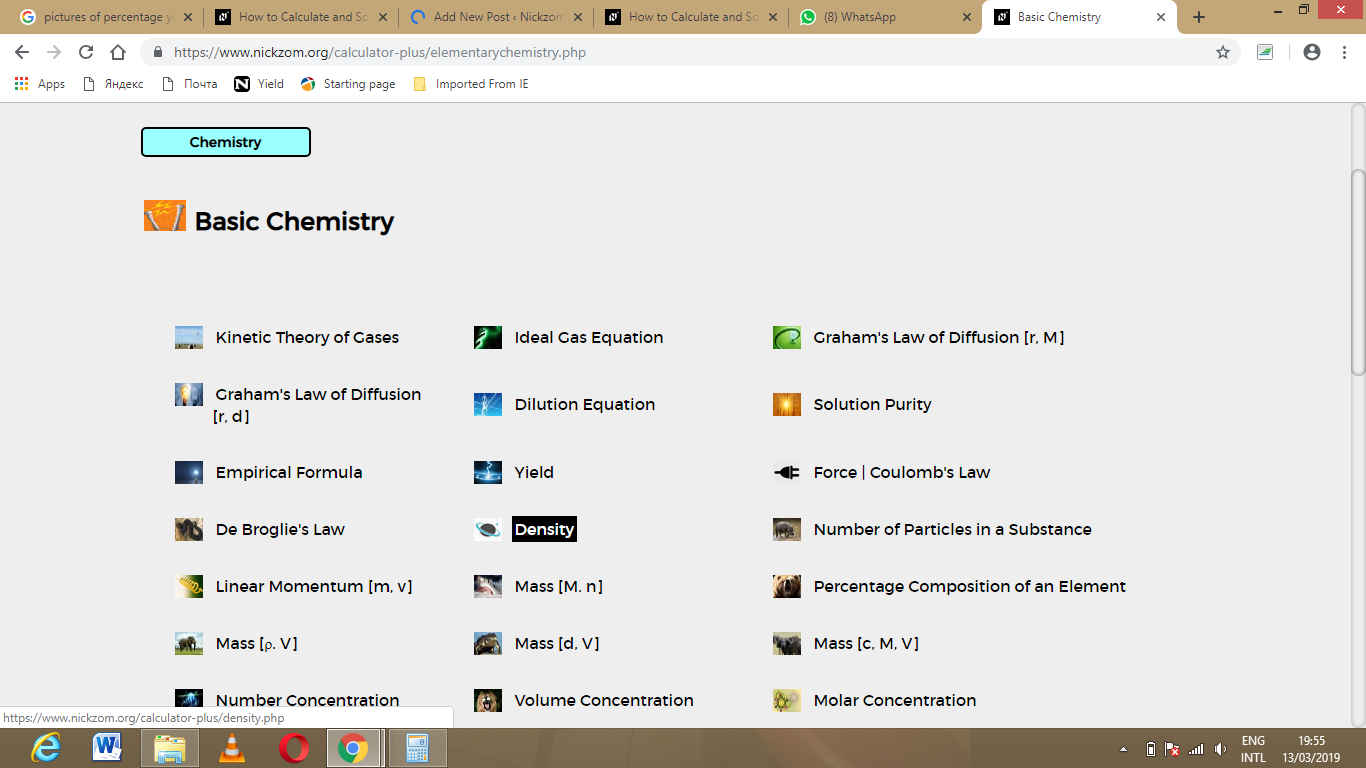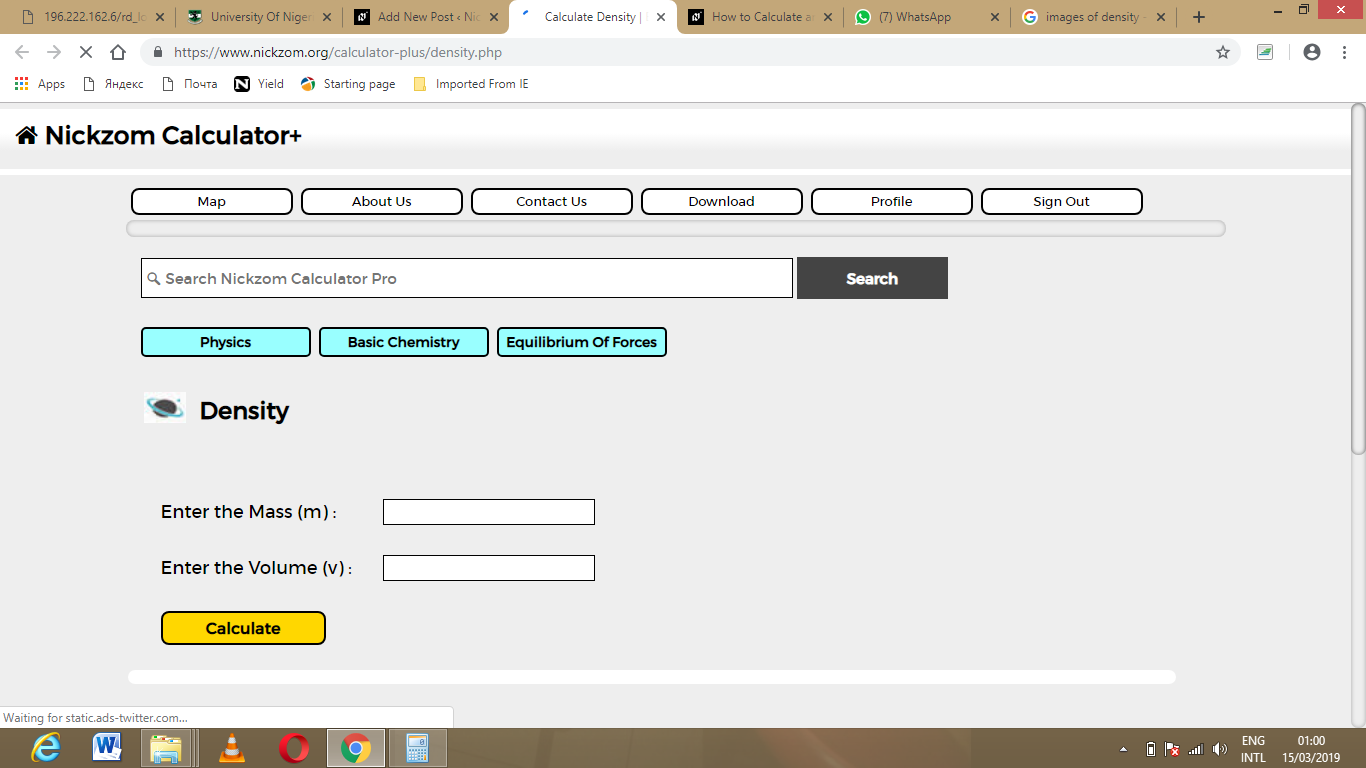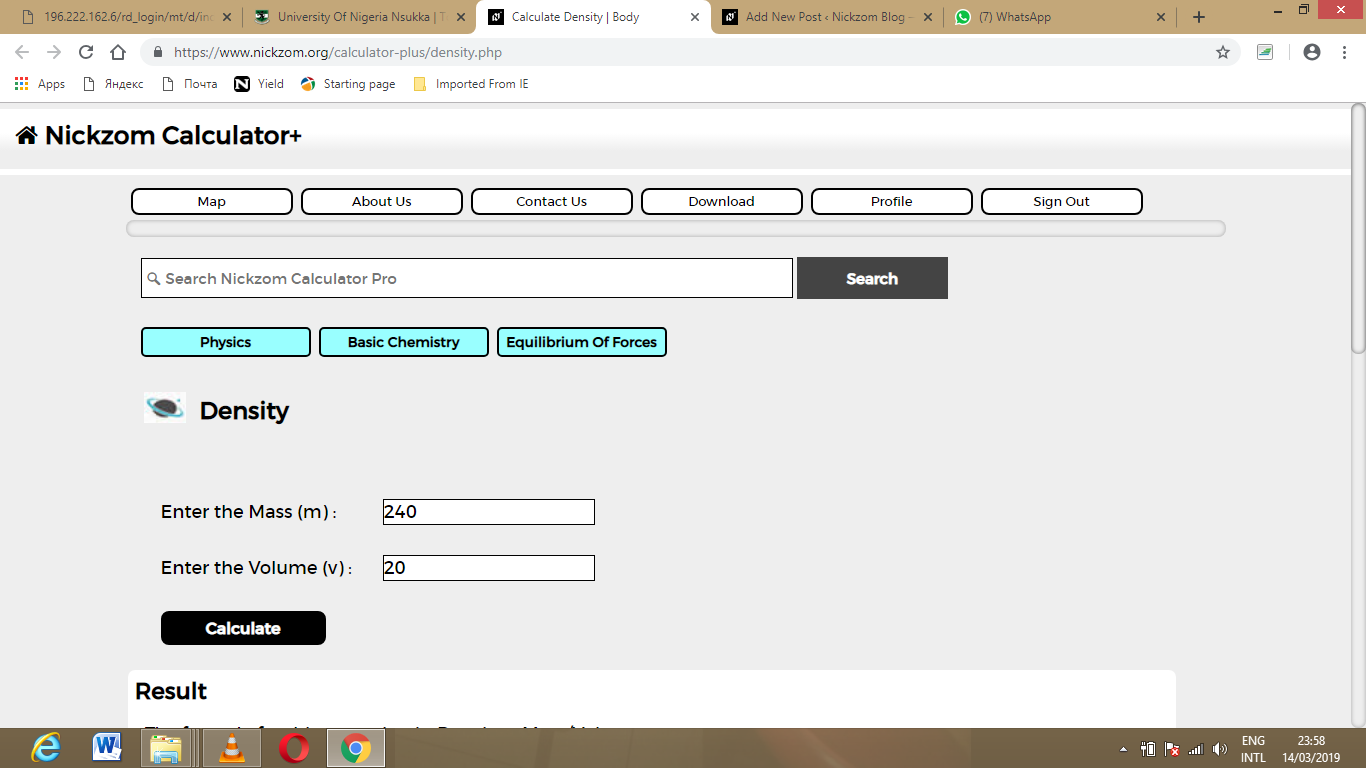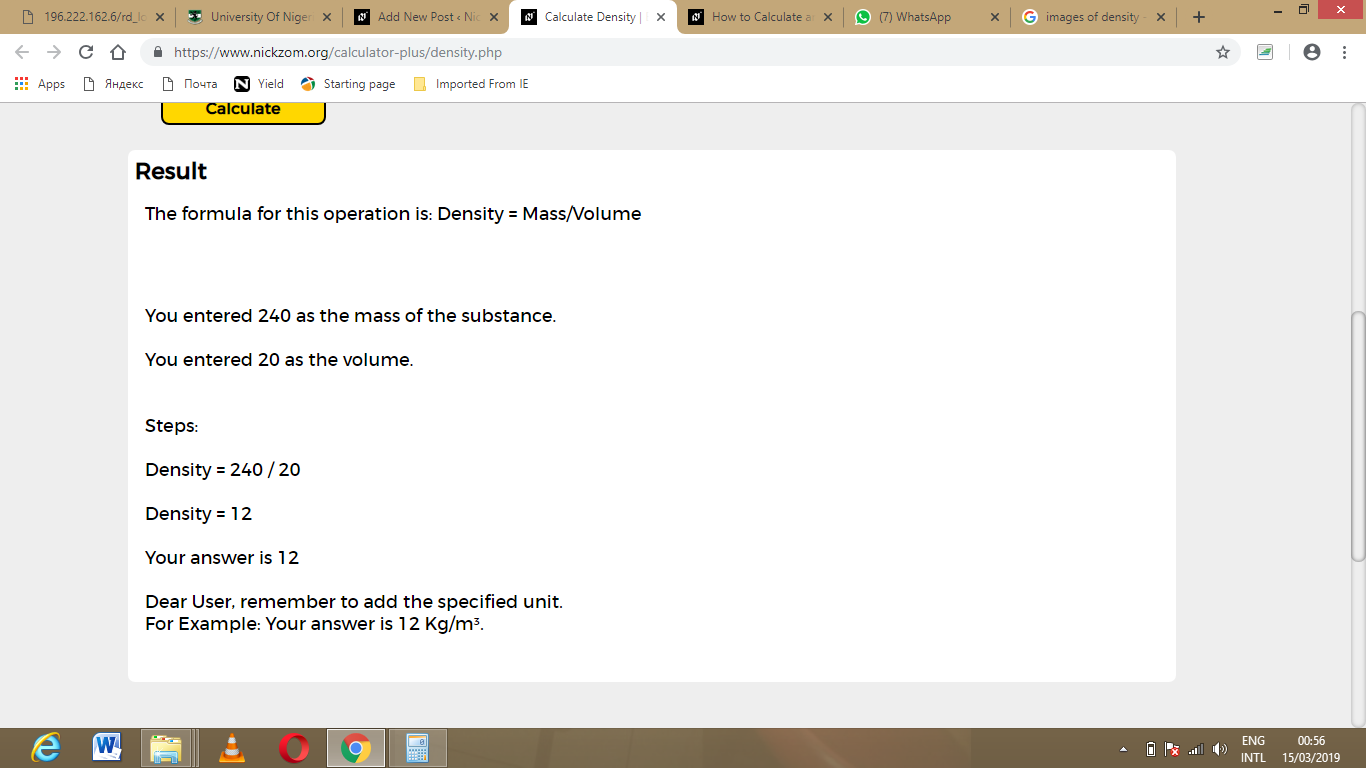# How to Calculate and Solve for Mass, Volume and Density | The Calculator EncyclopediaThe image above represents density.

To compute for the density, two essential parameters are needed and these parameters are mass (m) and volume (v).

The formula for calculating density:

Density = mass / volume

Let’s solve an example;
Given that the volume is 20 m³ with a mass of 240 kg. Find the density?

This implies that;
Volume = 20
Mass = 240

Density = mass / volume
Density = 240 / 20
Density = 12

Therefore, the density is 12 Kg/m³.

Calculating the Mass when the Density and Volume is Given.

Mass = Volume x Density

Let’s solve an example;
With a density of 90 kg/m³ and a volume of 15 m³, Find the mass?

This implies that;
Density = 90
Volume = 15

Mass = Volume x Density
Mass = 15 x 90
Mass = 1350

Therefore, the mass is 1350 kg.

Calculating the Volume when Mass and Density is Given.

Volume = mass / density

Let’s solve an example;
Find the volume with a mass of 50 kg and a density of 70 kg/m³?

This implies that;
Density = 70
Mass = 50

Volume = mass / density
Volume = 50 / 70
Volume = 0.714

Therefore, the volume is 0.714 m3.

Nickzom Calculator – The Calculator Encyclopedia is capable of calculating the density.

To get the answer and workings of the density using the Nickzom Calculator – The Calculator Encyclopedia. First, you need to obtain the app.

You can get this app via any of these means:

To get access to the professional version via web, you need to register and subscribe for NGN 1,500 per annum to have utter access to all functionalities.
You can also try the demo version via https://www.nickzom.org/calculator

Once, you have obtained the calculator encyclopedia app, proceed to the Calculator Map, then click on Basic Chemistry under ChemistryNow, Click on Density under Basic ChemistryThe screenshot below displays the page or activity to enter your values, to get the answer for the density according to the respective parameters which are the mass (m) and volume(v).Now, enter the values appropriately and accordingly for the parameters as required by the mass (m) is 240 and volume (v) is 20.Finally, Click on CalculateAs you can see from the screenshot above, Nickzom Calculator – The Calculator Encyclopedia solves for the density and presents the formula, workings and steps too.